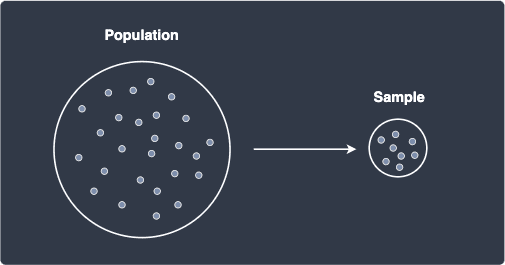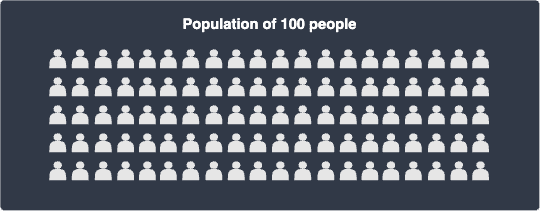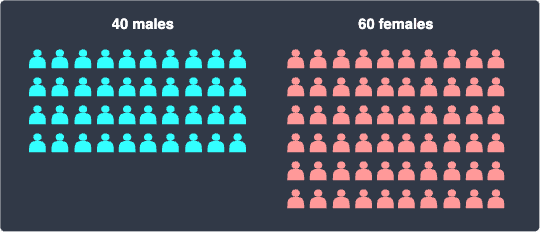search
Search
Unlock 100+ guides
search toc
close
account_circle
Profile
exit_to_app
Sign out
search
keyboard_voice
close
Searching Tips
Search for a recipe:
"Creating a table in MySQL"
Search for an API documentation: "@append"
Search for code: "!dataframe"
Apply a tag filter: "#python"
Useful Shortcuts
/ to open search panel
Esc to close search panel
to navigate between search results
d to clear all current filters
Enter to expand content previewDoc SearchCode Search BetaSORRY NOTHING FOUND!
mic
Start speaking...Voice search is only supported in Safari and Chrome.
Shrink
Navigate to

# Population, samples and sampling techniques

schedule Aug 12, 2023
Last updated
local_offer
Probability and Statistics
Tags
mode_heat
Master the mathematics behind data science with 100+ top-tier guides
Start your free 7-days trial now!

# Population and samples

In statistics, population refers to the entire set of people or objects of interest. For instance, a population of some study may be:

• all male adults of a country.

• all university graduates of 2022 in Japan.

• all vitamin C pills manufactured by a specific pharmaceutical company.

We are often interested in knowing some characteristics of the population. For instance, we might want to know the mean height of males so that we can understand some demographic trends. However, in most cases, because of budget, time or resource constraints, we are unable to obtain these population characteristics. Clearly, asking every male adult in the country is impossible. In rare cases when the population data is available, we have a so-called census.

Instead of gathering the entire population's data, we collect data from a small subset, or samples, of the population. For instance, samples of a study may be:

• a random selection of $500$ male adults in a country.

• a random selection of $100$ university graduates of 2022 in Japan.

• a collection of every $10$ vitamin C pills manufactured by the pharmaceutical company.

The relationship between population and sample is illustrated below:We then use the sample to draw conclusions (or make inferences) about the population. For instance, we might use the mean height of a sample of $500$ random male adults to infer that the mean height of the country's male adults is similar.

NOTE

An individual value in a sample of values is called an observation. For instance, suppose we asked three random adult males for their height. The sample might consist of the following observations:

$$160,180,170$$

# Sampling techniques

Whenever we make such inferences using a sample, we must ensure that the samples are representative of the targeted population. For instance, suppose we are interested in knowing the average salary of fresh graduates. If our sample consists only of graduates from the top university, then their salary is not reflective of all graduates - we will probably be overestimating the population mean salary of fresh graduates. In such cases when our sample is not representative of the targeted population, we say that our sample or sampling technique is biased.

We must therefore ensure that our sampling technique generates samples that reflect the underlying population. In this section, we will discuss four common sampling techniques:

• random sampling.

• stratified sampling.

• convenience sampling.

• voluntary response sampling.

## Random sampling

Random sampling is by far the simplest sampling technique that involves randomly selecting a subset from the population. For instance, consider the following population of $100$ people:Suppose we wanted to infer the mean height of this population using a sample of size $10$. For random sampling, we randomly select $10$ people from this population to form a single sample.

As discussed earlier, we want our samples to be representative of the underlying population. By forming a sample of random observations, we can ensure our own biases are not manifested in the data collection procedure.

Many statistical studies opt for random sampling because of its simplicity, but this sampling technique does have some drawbacks. Suppose our population of $100$ people consisted of $40$ males and $60$ females:Examples of our random sample of size $10$ might be as follows:

Number of males

Number of females

$5$

$5$

$9$

$1$

$0$

$10$

Can we say that a sample consisting of no males and all females is representative of the population? Definitely not - males are underrepresented and females are overrepresented. This is particularly a problem for our study because males are generally taller than females. Therefore, using a sample containing all females will underestimate the population height. To get around this problem, we can opt for another sampling technique called stratified sampling.

## Stratified sampling

Stratified sampling involves:

1. stratifying (or dividing) the population based on some trait. Each divided group is called a stratum.

2. randomly sampling from each stratum. The sample size of each random sample should be proportional to the size of the stratum.

3. combining the random samples to form a single sample.

Let's now go through an example - consider a population of $100$ people and we want to generate a sample of size $10$. Let's first stratify this population based on gender:Here, we have two strata - this word is just the plural of stratum. We now randomly sample from each stratum, but the key is to make their sample size proportional to the size of the stratum, which means that:

• since there are $40$ males, we randomly sample $4$ males.

• since there are $60$ females, we randomly sample $6$ females.

Finally, we combine the two random samples to form a single sample, which will be used for statistical inference. Unlike random sampling, stratified sampling guarantees that our sample consists of traits that are representative of the underlying population.

## Convenience sampling

Convenience sampling, as the name suggests, prioritizes convenience over the goal of generating a representative sample. For instance, suppose we just graduated from a top-tier university and wish to know the average salary of fresh graduates. For convenience, we form a sample consisting of our classmates, and estimate the average salary based on this sample.

The problem with this is that our classmates also graduated from the same top-tier university, so their salary will not be representative of the salary of all fresh graduates. Convenience sampling is perhaps the fastest and cheapest sampling technique, but we must be wary of drawing conclusions about the entire population.

## Voluntary Response Sampling

Voluntary response samples consist of individuals who volunteer to be part of the sample. For instance, suppose we own a product and wanted to find out what our customers think about the product. We decide to share a survey on our website and our customers can voluntarily decide whether to fill out the survey or not.

Chances are that only customers who feel strongly (either positively or negatively) about our product will fill out the survey and most of the users who feel neutral about our product will not bother. Therefore, the sample will likely consist of users with rather extreme opinions, which means that the sample is not representative of our entire customer base. Therefore, we should not generalize the survey results to our customer base.

WARNING

Convenience and voluntary response sampling are biased sampling techniques because the resulting sample is not representative of the underlying population.

# Implementing random and stratified sampling in Python

Consider the following Pandas DataFrame that represents a population of people:

 import pandas as pddf = pd.DataFrame({ 'name': ['a','b','c','d','e','f','g','h','i','j','k','l'], 'gender': ['M','M','M','M','F','F','F','F','F','F','F','F']})df.head() name gender0 a M1 b M2 c M3 d M4 e F 

This population consists of $12$ people of whom $4$ are male and $8$ are female.

## Random sampling

To randomly sample $3$ people from this population:

 n = 3df.sample(n, random_state=42) # returns a new Pandas DataFrame name gender10 k F9 j F0 a M 

Here, the random_state is a seed for reproducibility - running this code block will always result in the same random sample.

## Stratified sampling

Suppose we wanted to stratify (divide) the population based on gender and then generate a random sample of size $3$. Since the population is composed of $4$ males and $8$ females, our random sample should be composed of $1$ male and $2$ females to respect the proportions.

Here's the code to perform this stratified sampling:

 n = 3def get_df_prop(df_stratum): n_rows = len(df_stratum) # get the total number of rows (e.g. 4 for the male df) prop = n_rows / len(df) # get the proportions (e.g. 0.3333 for the male df) count = int(n * prop) # get the number of rows to extract (e.g. 1 for the male df) return df_stratum.sample(n=count, random_state=42)df.groupby('gender').apply(lambda df_stratum: get_df_prop(df_stratum)) name gendergender F 5 f F 9 j FM 1 b M 

Note the following:

• we first stratify our population based on gender using groupby('gender').

• we then use the apply(~) method, which iteratively takes in as argument a stratified DataFrame.

• our custom method get_df_prop(~) returns a random sample from the stratified DataFrame. The size of the random sample will be proportional to the size of the strata.

thumb_up
thumb_down
Comment
Citation
Ask a question or leave a feedback...
thumb_up
3
thumb_down
0
chat_bubble_outline
0
settings
Enjoy our search
Hit / to insta-search docs and recipes!